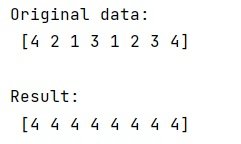# How to keep a running maximum of a NumPy array?

Learn, how can we keep a running maximum of a NumPy array in Python? By Pranit Sharma Last updated : March 30, 2023

## Running maximum of NumPy array values

Suppose that we are given a numpy array with 10 elements and we need to keep running the elements of this array. To keep a running maximum of a NumPy array, we can simply do this using a for loop but in the case of a large array, it will consume more time and space.

Hence, we will use numpy.ufunc.accumulate() which is used to accumulate the result of applying the operator to all elements.

For a multi-dimensional array, accumulate is applied along only one axis so repeated use is necessary if one wants to accumulate over multiple axes.

Let us understand with the help of an example,

## Python code for running maximum of NumPy array values

```# Import numpy
import numpy as np

# Creating a numpy array
arr = [4,2,1,3,1,2,3,4]

# Display original data
print("Original data:\n",arr,"\n")

# Using maximum accumulate
res = np.maximum.accumulate(arr)

# Display result
print("Result:\n",res)
```

### Output